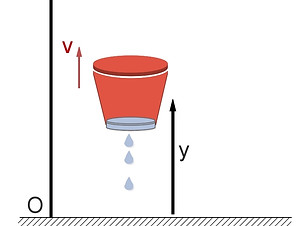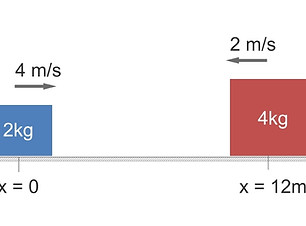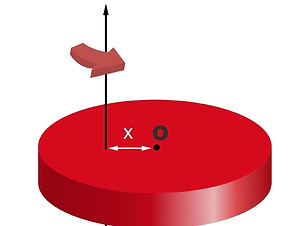top of page### PHYSICS PREP

Understanding the concepts in the field of Physics requires using them in solving problems. Here, we present  solutions to Physics problems from various topics. First year college and AP Physics students will find this resource extremely useful.

# <<< GO BACK TO THE MAIN BLOG PAGE#### Vectors

Vectors, Addition and Subtraction of Vectors, Dot (Scalar) Product, Cross (Vector) Product, Coordinate Rotations#### Work - Energy

Power, work, kinetic energy, gravitational potential energy, conservation of energy, work in three dimensions#### Oscillations

Harmonic oscillation, pendulum and spring motions, standing waves, sound waves, interference of waves#### Thermodynamics

Heat transfer, ideal gas law, kinetic theory of gases, PV diagrams, Entropy, four special processes#### Electric Circuits

Ohm's Law, DC and AC circuits, Kirchhoff's Rule, capacitors, RC circuits, combinations of electric components#### Kinematics

Projectile motion, constant acceleration, variable acceleration, velocity versus speed, circular motion, polar coordinates#### Collisions

System of particles, center of mass, impulse, conservation of linear momentum#### Statics

Systems in Equilibrium are investigated. The knowledge of torque and force is being applied here.#### Electricity

Electric Force, electric field and potential, equipotential surfaces and lines, energy density, Gauss' Law, electric energy#### Optics

Lenses, mirrors, polarization, single slit and double slit experiments, index of refraction, Snell's Law, thin films#### Dynamics

Newton's Laws, physical forces, inclined plane motion, friction, Hooke's Law, weight, linear momentum#### Rotation

Rigid bodies, angular momentum, rotation about a fixed axis, torque, conservation of angular momentum, rolling motion#### Fluid Mechanics

Types of fluids, static fluids, buoyancy force, fluid dynamics, continuity and Bernoulli's equations#### Magnetism

Magnetic fields, magnetic force, electromagnetic induction, magnetic flux, Ampere's Law, particle motions

bottom of page